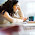## Thursday, November 4, 2010

### Which cones are needed to represent almost all convex optimization problems?

Recently I visited my Professor Yinyu Ye at Stanford university.  I worked with during my ph.d. studies where we did some nice work together. Now we hope we might have some new ideas to explore. More about that later. During my visit I also talked Stephen Boyd and Jacob Mattingley. Jacob also gave me a presentation of his interesting ph.d. work which he defended while I was at Stanford.

During a lunch with Yinyu, Stephen and some other Standford guys Stephen said something like: "Almost all convex optimization problem can be formulated using a combination of  linear, quadratic, semi-definite and the exponential cones". It is a view I am sharing. Given it is true it has an important practical ramification I will return to shortly. Stephen is aware that his statement is hard to prove but I think it is true like almost all large scale LPs are sparse. It is not something  that is not universally true but it is true in practice.

Please note that any convex problem can be formulated in conic form but Stephens postulate that only very few cone types are require. Moreover, currently it is only the exponential cone we do not know how to deal with in practice because it is a so-called nonsymmetric cone.

Occasionally at MOSEK support we get a question like: "I have a nonlinear model. How do I solve it with MOSEK?"  My first reply is always: "Is your model convex?" because MOSEK only deals with convex models. If the user knows what convexity is then user will usually reply yes. Maybe, the user will add that it looks complicated to solve general convex convex models with MOSEK. That is particularly true if you do not use a modeling language like AMPL or GAMS. Here comes Stephen Boyds observation handy because if your model does not include exponential terms (or logarithmic terms) or semi-definite terms then most likely the problem can be formulated as a conic quadratic optimization problem. That is fortunate because
• conic quadratic optimization problems are as easy as linear problems to deal with software wise. The user does not have to bother with gradients and Hessians for instance.
• the optimizer for conic quadratic optimization problems is much more robust than the optimizer for general convex optimization problems.
Recently after I came back from Stanford an user wrote to MOSEK  support: "I have this complicated convex problems that cannot be formulated as conic quadratic optimization problem. How should I solve it with MOSEK?" After some back and forth I got him to reveal that it essentially had nonlinear functions of the type

max(x,0)^2 <= t

Now that is in fact conic quadratic representable as follows

s^2 <= t
x    <= s

So, it turned that the complicated convex model is a conic quadratic representable contrary to the initial statement.

The upshot from Stephen Boyds statement is that if your model is convex and does not include exponential or logarithmic terms then is very likely it can represented by conic quadratic or a semi-definite problem. I think that is a very useful observation in practice.

PS. Usually when reformulating a problem as conic quadratic optimization problem then the number of constraints and variables are expanded. Some thinks that inefficient. However, it should be noted that the structure introduced is very sparse and hence does not hurt performance much.  There even some cases (thinks QPs with a low rank dense Hessian) where the conic quadratic representation is bug but requires much less space when stored sparse.

#### 1 comment:

1.Well explained .Keep updating Artificial Intelligence Online Training